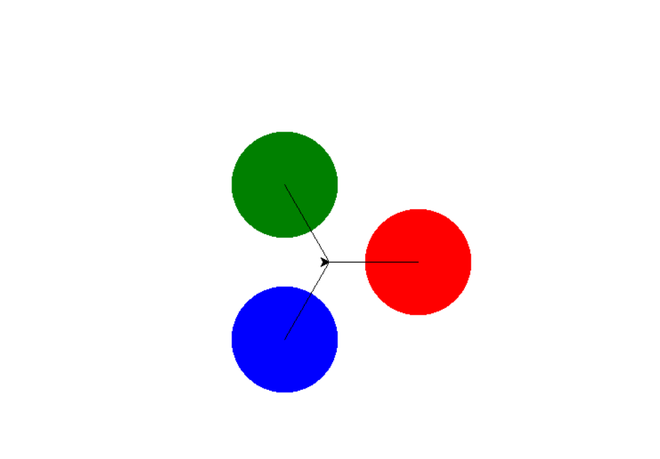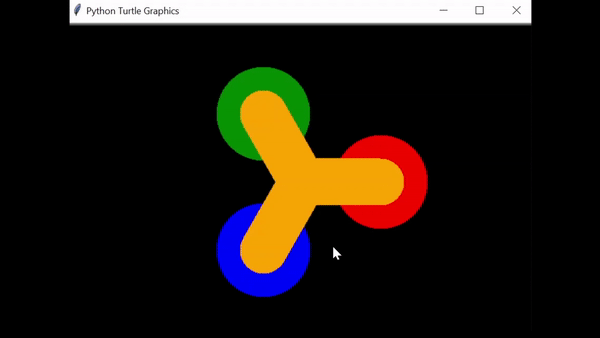# Fidget Spinner using Python

• Last Updated : 30 Jun, 2021

Prerequisite: Turtle Programming in Python

In this article, we are going to create Fidget Spinner using the Python turtle module. It is a Python feature like a drawing board, which lets us command a turtle to draw all over it! We can use functions like turtle.forward() and turtle.right() which can move the turtle around.

#### Let’s see the stepwise implementation:

Step 1: Drawing the fidget spinner

Here in this piece of code, we will initialize the state of the fidget spinner, angles for rotating it in both clockwise and anticlockwise direction and make the colored graphics required to make the fidget spinner.

## Python3

 `# initial state of spinner is null (stable) ` `state``=` `{``'turn'``:``0` `} ` ` `  `# Draw fidget spinner ` `def` `spin(): ` `    ``clear() ` ` `  `    ``# Angle of fidget spinner ` `    ``angle ``=` `state[``'turn'``]``/``10` `    ``right(angle) ` ` `  `    ``# move the turtle forward by specified ` `    ``# distance ` `    ``forward(``100``) ` ` `  `    ``# draw a dot with diameter 120 using colour  ` `    ``# red ` `    ``dot(``120``, ``'red'``) ` ` `  `    ``# move the turtle backward by specified  ` `    ``# distance ` `    ``back(``100``) ` ` `  `    ``"second dot"` `    ``right(``120``) ` `    ``forward(``100``) ` `    ``dot(``120``, ``'blue'``) ` `    ``back(``100``) ` ` `  `    ``"third dot"` `    ``right(``120``) ` `    ``forward(``100``) ` `    ``dot(``120``, ``'green'``) ` `    ``back(``100``) ` `    ``right(``120``) ` ` `  `    ``update() `

Output:Step 2: Animating the fidget spinner

In this step, we will call a function animate() which will animate the fidget spinner by seeing if the state is greater than 0 then decrement one from it decreases and call the spin function again. After that, a timer is installed which will call the animate function again after 20 milliseconds

## Python3

 `# Animate fidget spinner ` `def` `animate(): ` `    ``if` `state[``'turn'``] > ``0``: ` `        ``state[``'turn'``] ``-``=` `1` ` `  `    ``spin() ` `    ``ontimer(animate, ``20``) `

Step 3: Moving the fidget spinner, setting up the window, and tracing the spinner back to its original position

Here we will define the flick function which will move the fidget spinner by increasing its state to 40, also we will set up a window and its background color, we will use a tracer that brings back the fidget spinner into its initial state after completing its rotation and after that, we will define the width and color of our fidget spinner and at last, we will define the key for moving the fidget spinner.

## Python3

 `# Flick fidget spinner ` `def` `flick(): ` `     `  `    ``# acceleration of spinner ` `    ``state[``'turn'``] ``+``=` `40` ` `  ` `  `# setup window screen ` `setup(``600``, ``400``, ``370``, ``0``) ` `bgcolor(``"black"``) ` ` `  `tracer(``False``) ` `'''tracer brings back the fidget spinner into its initial state ` `after completing the rotation'''` ` `  `# wing of fidget spinner ` `width(``60``) ` `color(``"orange"``) ` ` `  `# keyboard key for the rotation of spinner ` `onkey(flick, ``'space'``) ` ` `  `listen() ` `animate() ` `done() `

Below is the complete implementation:

## Python3

 `# import object from module turtle ` `from` `turtle ``import` `*` ` `  `# initial state of spinner is null (stable) ` `state``=` `{``'turn'``:``0` `} ` ` `  `# Draw fidget spinner ` `def` `spin(): ` `    ``clear() ` ` `  `    ``# Angle of fidget spinner ` `    ``angle ``=` `state[``'turn'``]``/``10` ` `  `    ``# To rotate in clock wise we use right ` `    ``# for Anticlockwise rotation we use left ` `    ``right(angle) ` ` `  `    ``# move the turtle forward by specified distance ` `    ``forward(``100``) ` ` `  `    ``# draw a dot with diameter 120 using colour red ` `    ``dot(``120``, ``'red'``) ` ` `  `    ``# move the turtle backward by specified distance ` `    ``back(``100``) ` ` `  `    ``"second dot"` `    ``right(``120``) ` `    ``forward(``100``) ` `    ``dot(``120``, ``'blue'``) ` `    ``back(``100``) ` ` `  `    ``"third dot"` `    ``right(``120``) ` `    ``forward(``100``) ` `    ``dot(``120``, ``'green'``) ` `    ``back(``100``) ` `    ``right(``120``) ` ` `  `    ``update() ` ` `  `# Animate fidget spinner ` `def` `animate(): ` `    ``if` `state[``'turn'``]>``0``: ` `        ``state[``'turn'``]``-``=``1` `     `  `    ``spin() ` `    ``ontimer(animate, ``20``) ` `     `  `# Flick fidget spinner ` `def` `flick(): ` `    ``state[``'turn'``]``+``=``40` `#acceleration of spinner ` ` `  `# setup window screen ` `setup(``600``, ``400``, ``370``, ``0``) ` `bgcolor(``"black"``) ` ` `  `tracer(``False``) ` ` `  `# wing of fidget spinner ` `width(``60``) ` `color(``"orange"``) ` ` `  `# keyboard key for the rotation of spinner ` `onkey(flick,``'space'``) ` ` `  `listen() ` `animate() ` `done() `

Output:My Personal Notes arrow_drop_up
Recommended Articles
Page :